# Get help with conic sections equations

Recent questions in Conic sectionskdgg0909gn 2021-11-20 Answered

### Given the force field F, find the work required to move an object on the given oriented curve. $$\displaystyle{F}={\left\langle{y},{x}\right\rangle}$$ on the parabola $$\displaystyle{y}={2}{x}^{{{2}}}{\mathfrak{{o}}}{m}{P}{S}{K}{\left({0},{0}\right)}$$ to $$\displaystyle{\left({2},{8}\right)}$$Cariglinom5 2021-11-18 Answered

### Determine the current flowing through an element if the charge flow is given by a) $$\displaystyle{q}{\left({t}\right)}={\left({3}{t}+{8}\right)}{m}{C}$$ b) $$\displaystyle{q}{\left({t}\right)}={\left({8}{t}^{{{2}}}+{4}{t}-{2}\right)}{C}$$ c) $$\displaystyle{q}{\left({t}\right)}={\left({3}{e}^{{-{1}}}-{5}{e}^{{-{2}{t}}}\right)}{n}{C}$$ d) $$\displaystyle{q}{\left({t}\right)}={10}{\sin{{120}}}\pi{t}\ {p}{C}$$ e) $$\displaystyle{q}{\left({t}\right)}={20}{e}^{{-{4}{t}}}{\cos{{50}}}{t}\mu{C}$$Rui Baldwin 2021-10-03 Answered

### The current in a wire varies with time according to the relation $$\displaystyle{I}={55}{A}?{\left({0.65}\frac{{A}}{{s^2}}{}\right)}{t}^{2}$$. How many coulombs of charge pass a cross section of the wire in the time interval between $$\displaystyle{t}={0}$$ and $$\displaystyle{t}={8.5}{s}$$ ? Express your answer using two significant figures. What constant current would transport the same charge in the same time interval? Express your answer using two significant figures.nicekikah 2021-08-15 Answered

### The current in a wire varies with time according to the relation $$\displaystyle{I}={55}{A}?(0.65\frac{A}{s^2}){t}^{2}$$. How many coulombs of charge pass a cross section of the wire in the time interval between $$\displaystyle{t}={0}$$ and $$\displaystyle{t}={8.5}{s}$$ ? Express your answer using two significant figures. What constant current would transport the same charge in the same time interval? Express your answer using two significant figures.smileycellist2 2021-08-15 Answered

### Sketch the following conic sections: $$\displaystyle{4}^{{{2}}}-{4}{y}+{8}-{4}{x}^{{{2}}}={0}$$Brittney Lord 2021-08-15 Answered

### Solve the system and graph the curves $$\begin{cases}x^{2}+3x-y+2=0 \\ y-5x=1 \end{cases}$$Carol Gates 2021-08-15 Answered

### Find the relation between the given quadric surfaces and conic sections.aortiH 2021-08-15 Answered

### To solve the given inequality and to write the solution set in interval notation.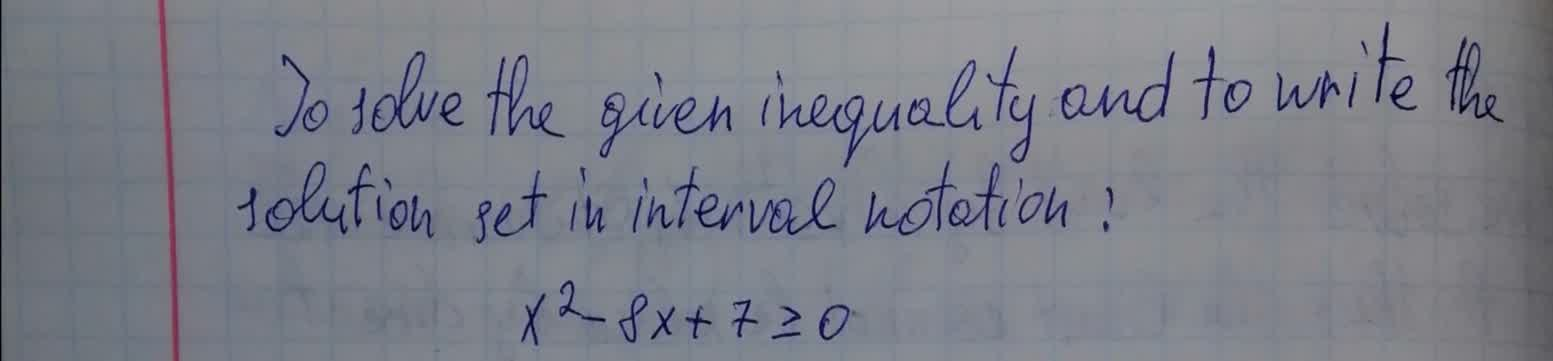ediculeN 2021-08-15 Answered

### To simplify: The given radical expression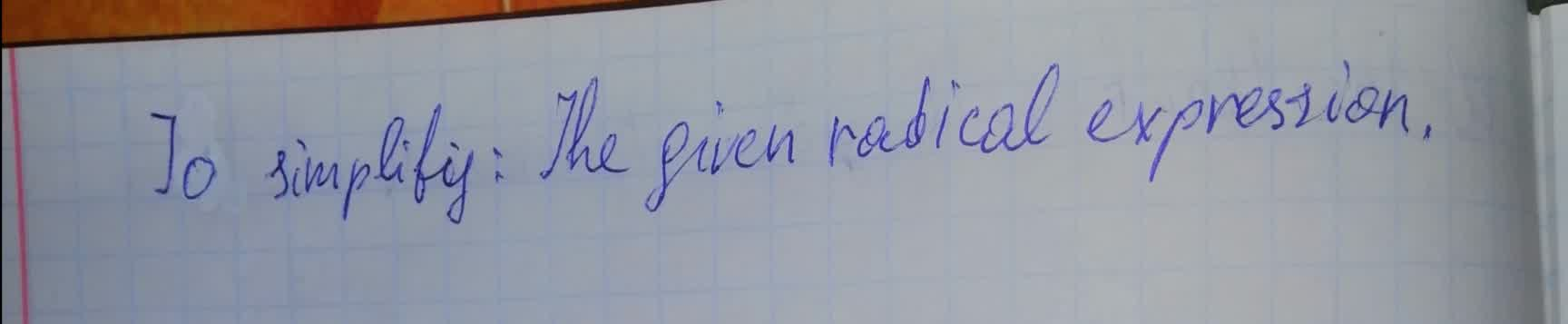Phoebe 2021-08-15 Answered

### To simplify the given expression.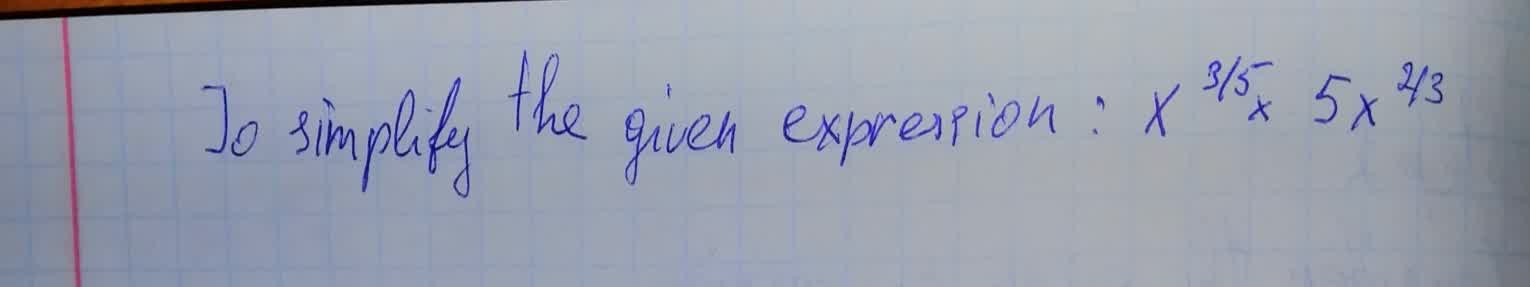Jaya Legge 2021-08-15 Answered

### To determine: The radical form of $$\sqrt{64}$$ in the rational exponent form.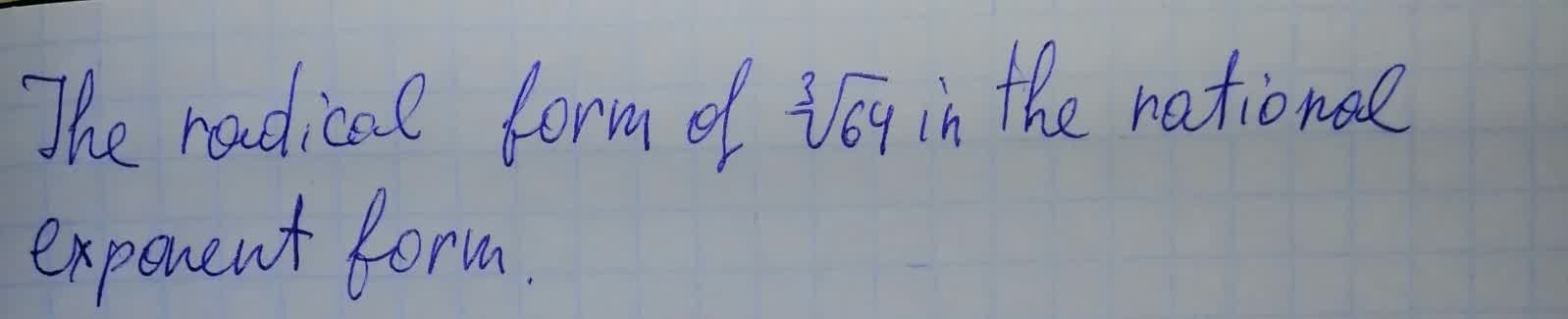arenceabigns 2021-08-15 Answered

### To calculate: The simplification of the expression $$\displaystyle{\frac{{{7}}}{{\sqrt{{{3}{x}}}}}}+{\frac{{\sqrt{{{3}{x}}}}}{{{x}}}}$$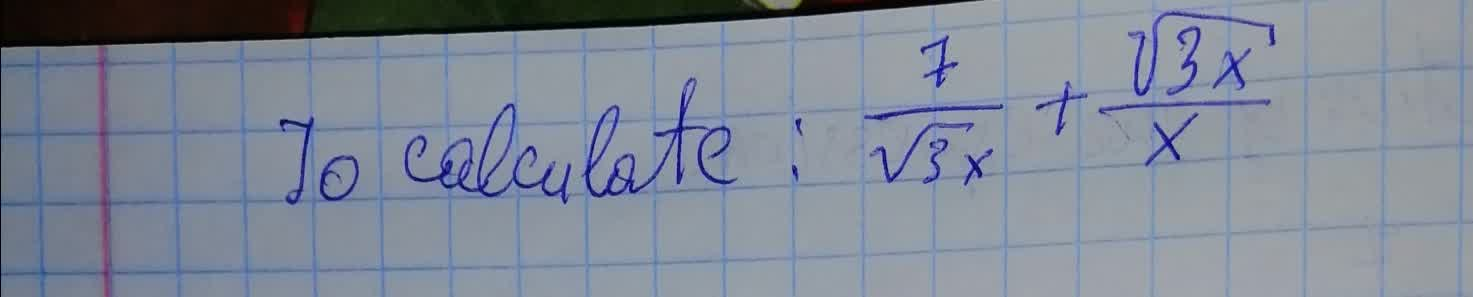Clifland 2021-08-15 Answered

### To calculate: The simplification of the expression $$\displaystyle{\frac{{\sqrt{{{50}}}}}{{\sqrt{{{x}-{2}}}}}}$$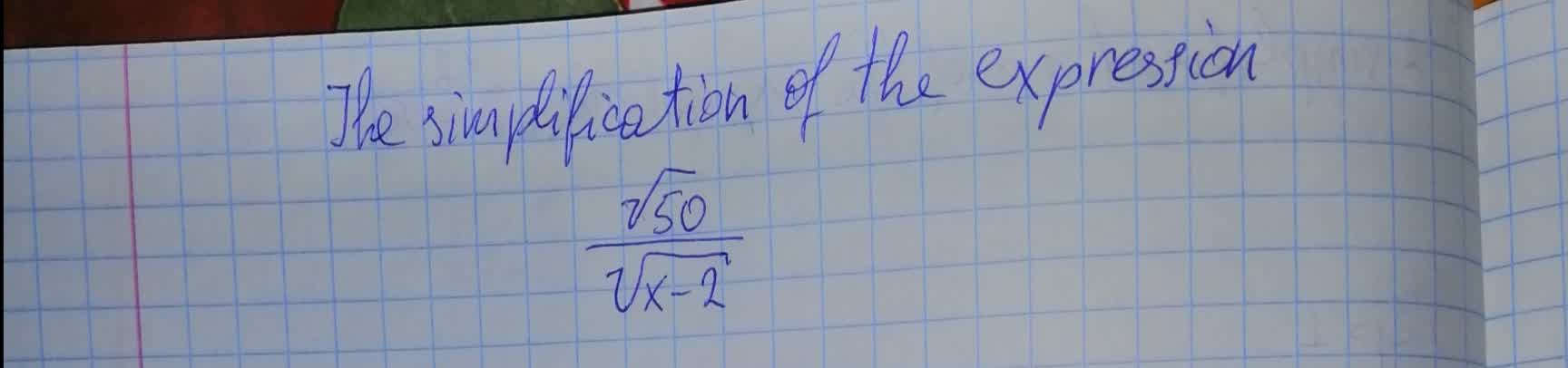boitshupoO 2021-08-15 Answered

### To calculate: The simplified form of the expression , $$\displaystyle{\frac{{{12}}}{{\sqrt{{{6}}}+\sqrt{{2}}}}}$$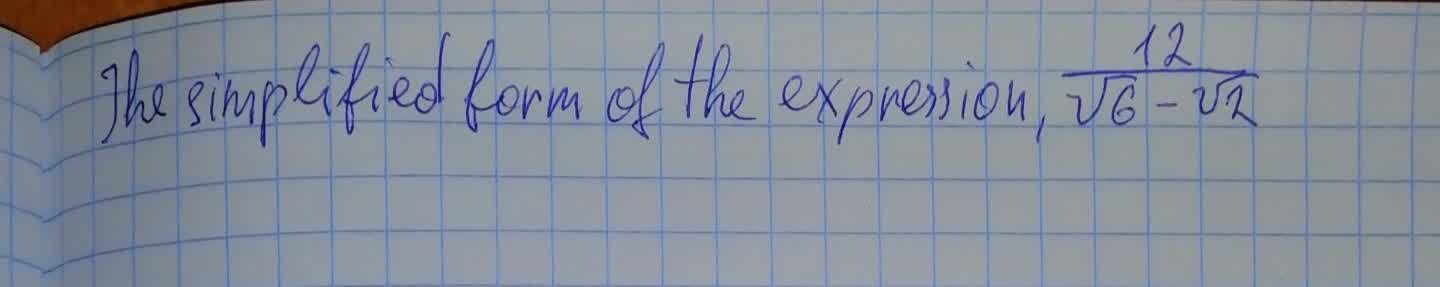Clifland 2021-08-14 Answered

### Give the eccentricities of conic sections with one focus at the origin along with the directrix corresponding to that focus. Find a polar equation for each conic section. 1) $$\displaystyle{e}={\frac{{{1}}}{{{4}}}},\ {x}=-{2}$$ 2) $$\displaystyle{e}={\frac{{{1}}}{{{3}}}},\ {y}={6}$$Chesley 2021-08-14 Answered

### Give the eccentricities of conic sections with one focus at the origin along with the directrix corresponding to that focus. Find a polar equation for each conic section. $$\displaystyle{e}=\frac{{1}}{{5}},\ {y}=-{10}$$Tazmin Horton 2021-08-14 Answered

### To identify: The conic represented by the following polar equation and graph it by using the graphing utility: $$\displaystyle{r}={\frac{{{8}}}{{{4}+{3}{\sin{\theta}}}}}$$Mylo O'Moore 2021-08-14 Answered

### Graph the lines and conic sections $$\displaystyle{r}={\frac{{{8}}}{{{4}+{\sin{\theta}}}}}$$shadsiei 2021-08-14 Answered

### Write the following equation in standard form and sketch its graph $$\displaystyle{9}{x}^{{{2}}}+{72}{x}-{64}{y}^{{{2}}}+{128}{y}+{80}={0}$$Ayaana Buck 2021-08-14 Answered

...